# Newman-Keuls (S-N-K)

The test statistic is: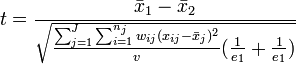$t=\frac{\bar x_1-\bar x_2}{\sqrt{\frac{\sum^{J}_{j=1}\sum^{n_j}_{i=1} w_{ij}(x_{ij} - \bar x_j)^2}{v}(\frac{1}{e_1}+\frac{1}{e_1})}}$

where: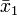$\bar x_1$ and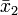$\bar x_2$ are the means of the two groups being compared and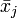$\bar x_j$ is the mean of the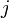$j$ of$J$ groups,
when applying the test to Repeated Measures, each respondent’s average is initially subtracted from their data and it is this corrected data that constitutes of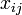$x_{ij}$,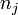$n_j$ is the number of observations in the$j$th of$J$ groups,$w_{ij}$ is the Calibrated Weight for the$i$th observation in the$j$ group,$e_j$ is the Effective Sample Size for the$j$ group,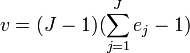$v = (J - 1)(\sum^J_{j=1} e_j - 1)$ for Repeated Measures and$v = \sum^J_{j=1} e_j - J$ otherwise.$t$ is evaluated using a Tukey’s Studentized Range distribution with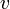$v$ degrees of freedom for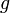$g$ groups, where$g$ is determined by a step down analysis (see Begun, Janet M.; Gabriel, K. Ruben, 1981, "Closure of the Newman-Keuls Multiple Comparisons Procedure", Journal of the American Statistical Association, 76, 241-245).